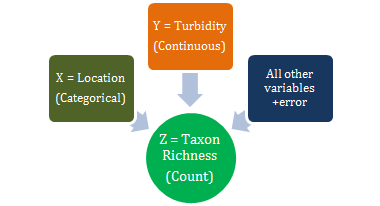# Inserting your own figures, graphs and tables

## SEB Inserting your own figures, graphs and tables

1. Be sure to cross reference your figures, graphs and tables in-text - see the 3 examples below.

2. Figures, graphs and tables must have captions - see the 3 examples below.

• Figures, images and graphs are labelled Figure, and the caption goes below the figure or graph;
• Tables are labelled Table, and the caption is inserted above the table.

3. If you are inserting figures, graphs and tables from other sources see the section Inserting figures (includes graphs) and tables from other sources.

4. Also see this article for examples of how figures, graphs and tables appear in a typical scientific article.

## Example 1: Figure

In-text of your document write eg Labelling allergens on a patient as shown in Figure 1 is useful.Figure 1: Conceptual model (z = response variable, x= location, y= turbidity).

## Example 2: Graph

In-text of your document write eg. Passenger numbers at London airports between 1990 and 2011 are shown in Figure 2 below.Figure 2: Passenger numbers for London Airports 1990-2011.

## Example 3: Table

In-text of your document write: Data sample specifications are shown in Table 1.

Table 1: Data sample specifications